# Slides & my Presentation style

this adds ?showNotes=true to the file

click this link for pdf printable + notes

HTML is best viewed in chrome

# Overview

• Repeated measures
• An example to guide you through the steps
• The mixed model
• Inference
• Assumption checking
• Shrinkage
• Terminology
• Convergence Problems
• Multiple random variables

## Repeated measures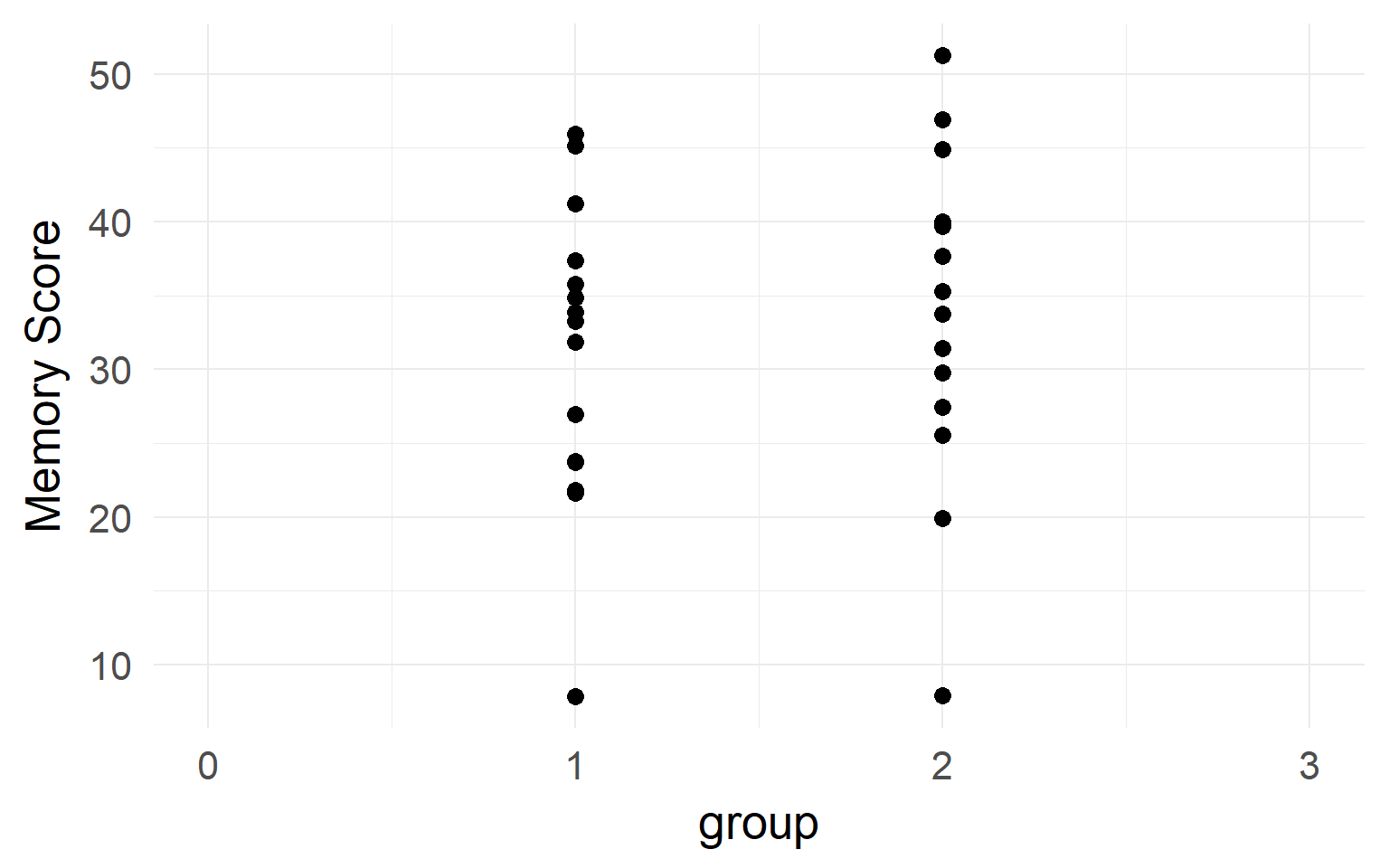It looks like there is no difference between groups?

But what if we have data from within the same subjects?

## Repeated measures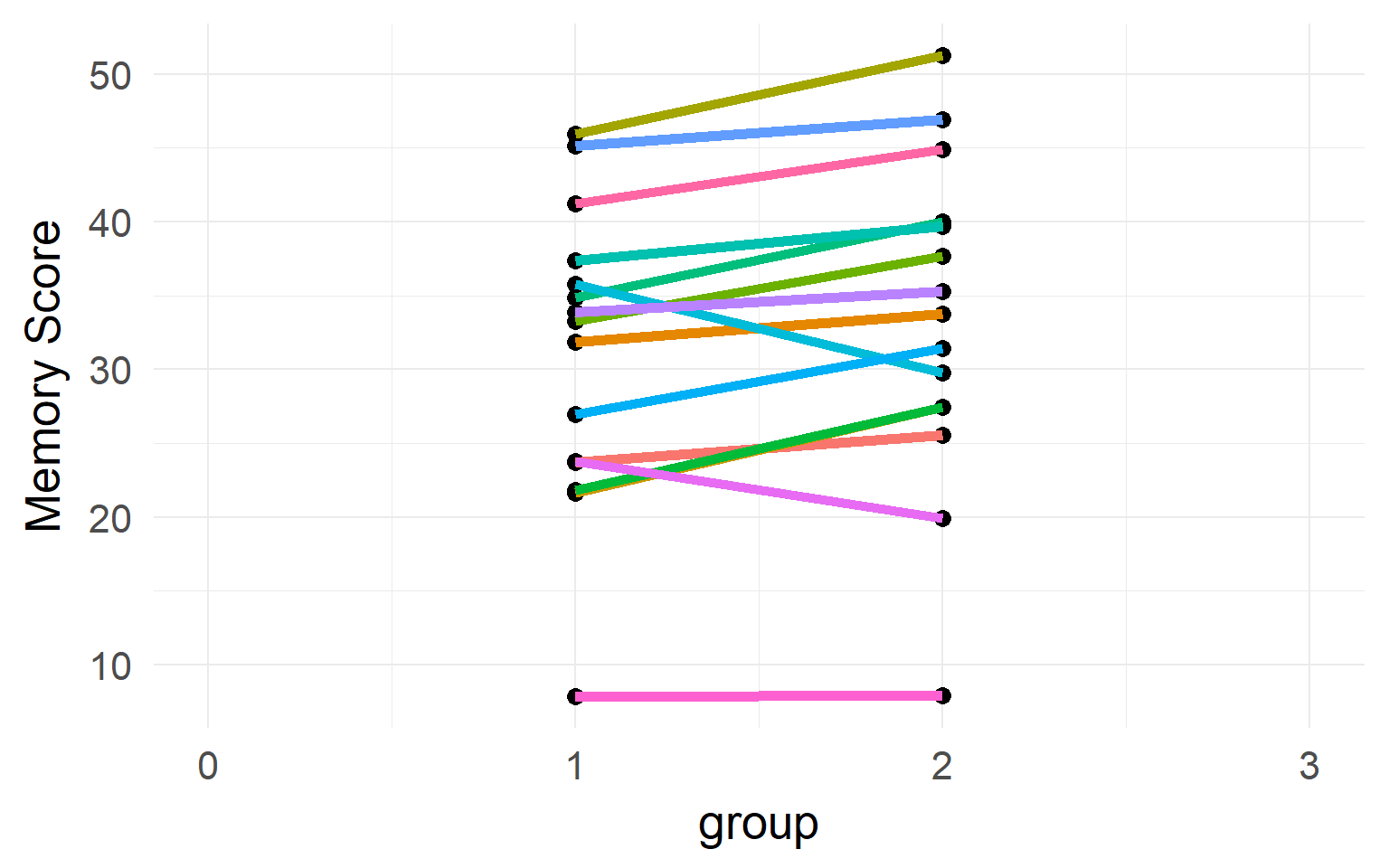## Individual differences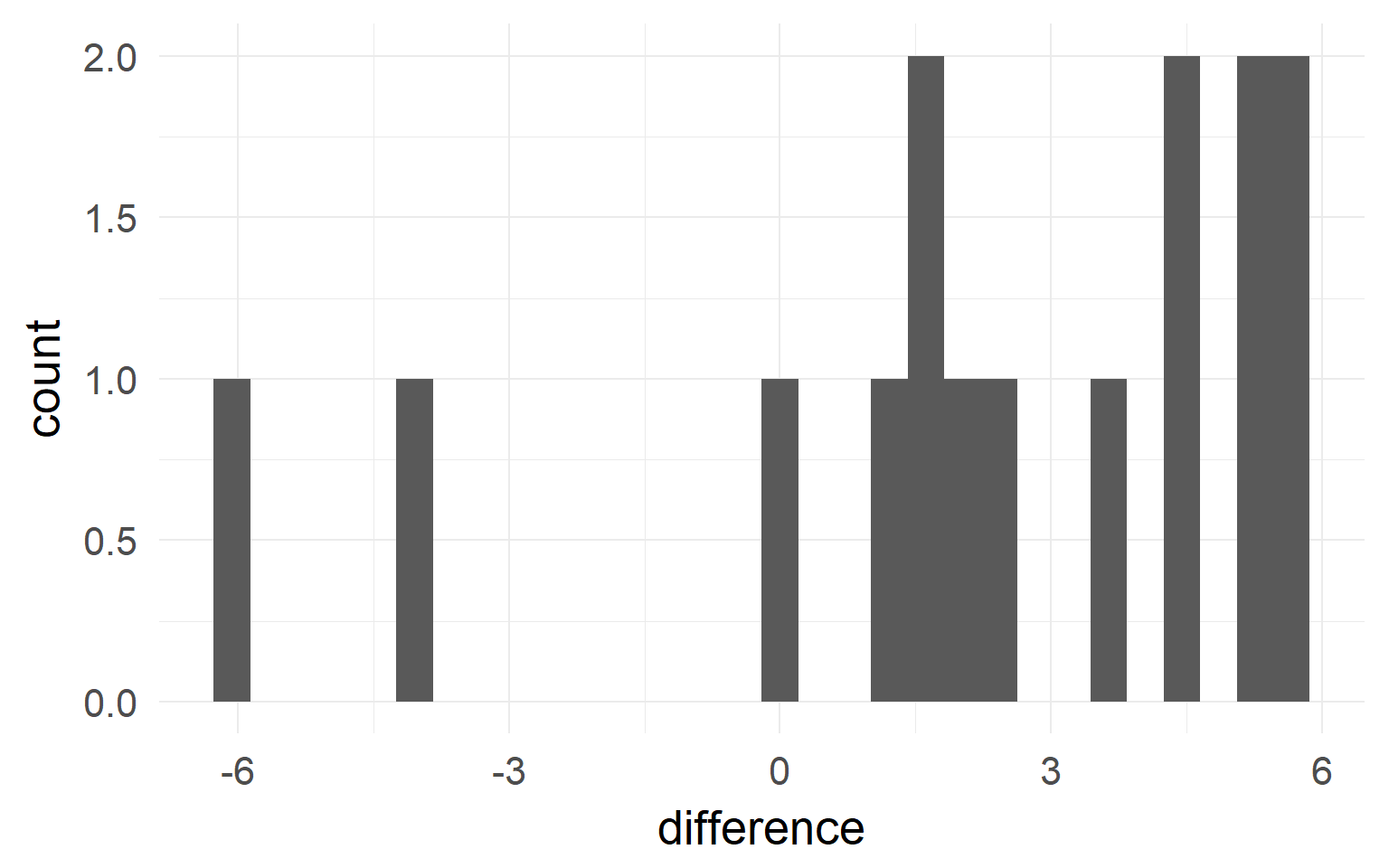Between-Subject variability can be removed by repeated measures.

Never analyze repeated measures data without taking repeated measures into account (Disaggregation, Pseudoreplication)

## Accouting for repeated measures?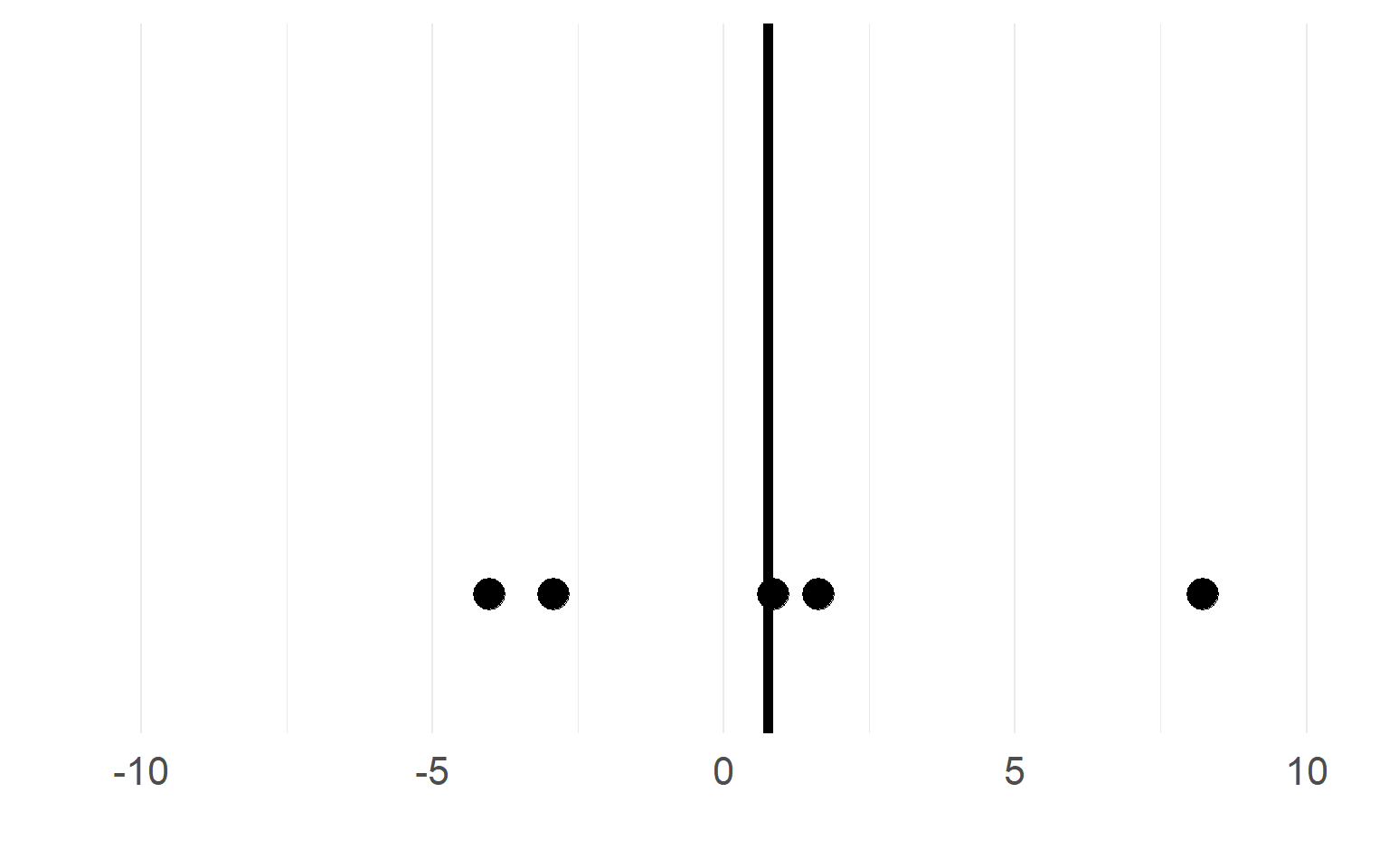Black = mean of the subject.

$$n_S=5$$ subjects

## Sampling distributions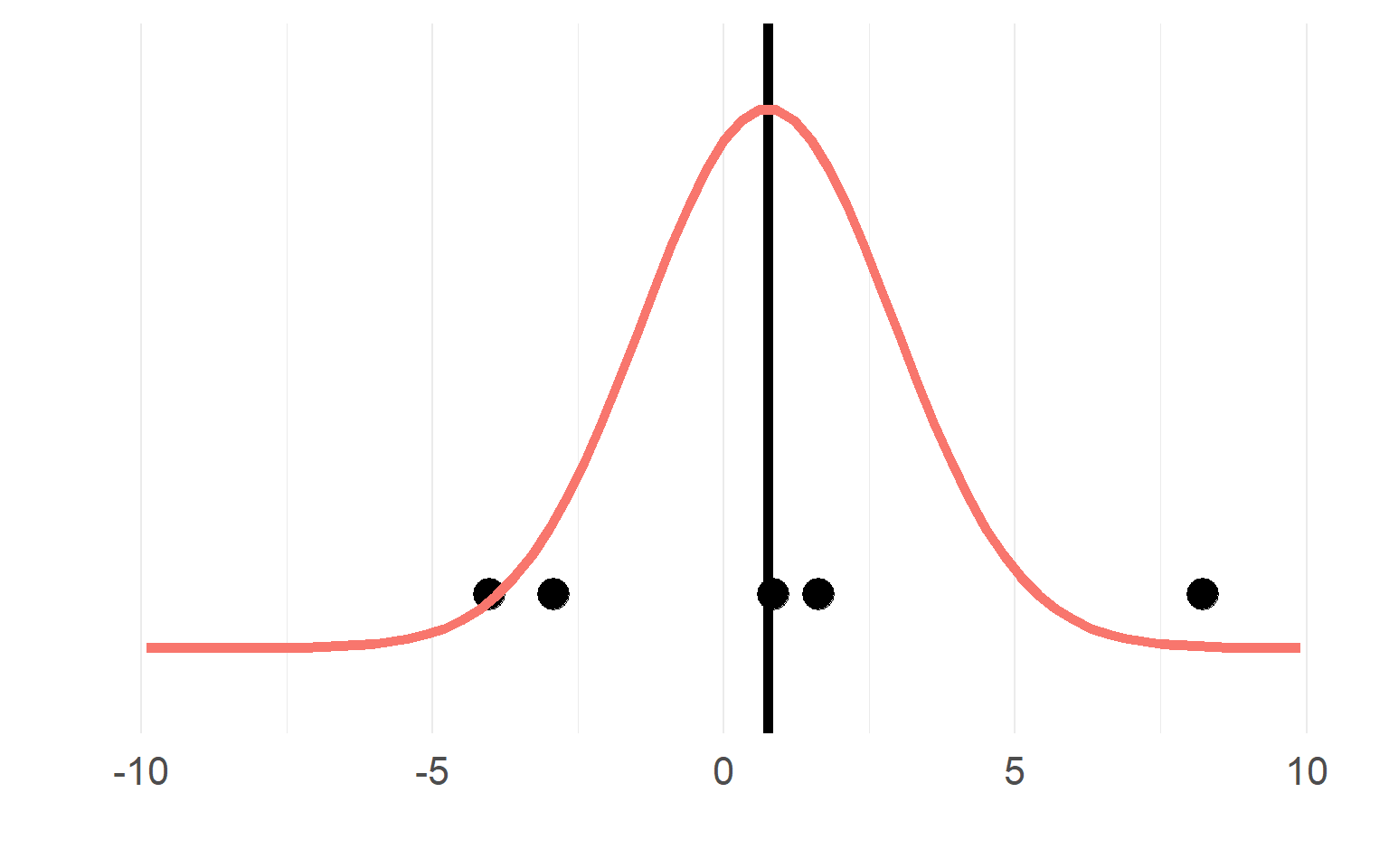## Multiple trials per subject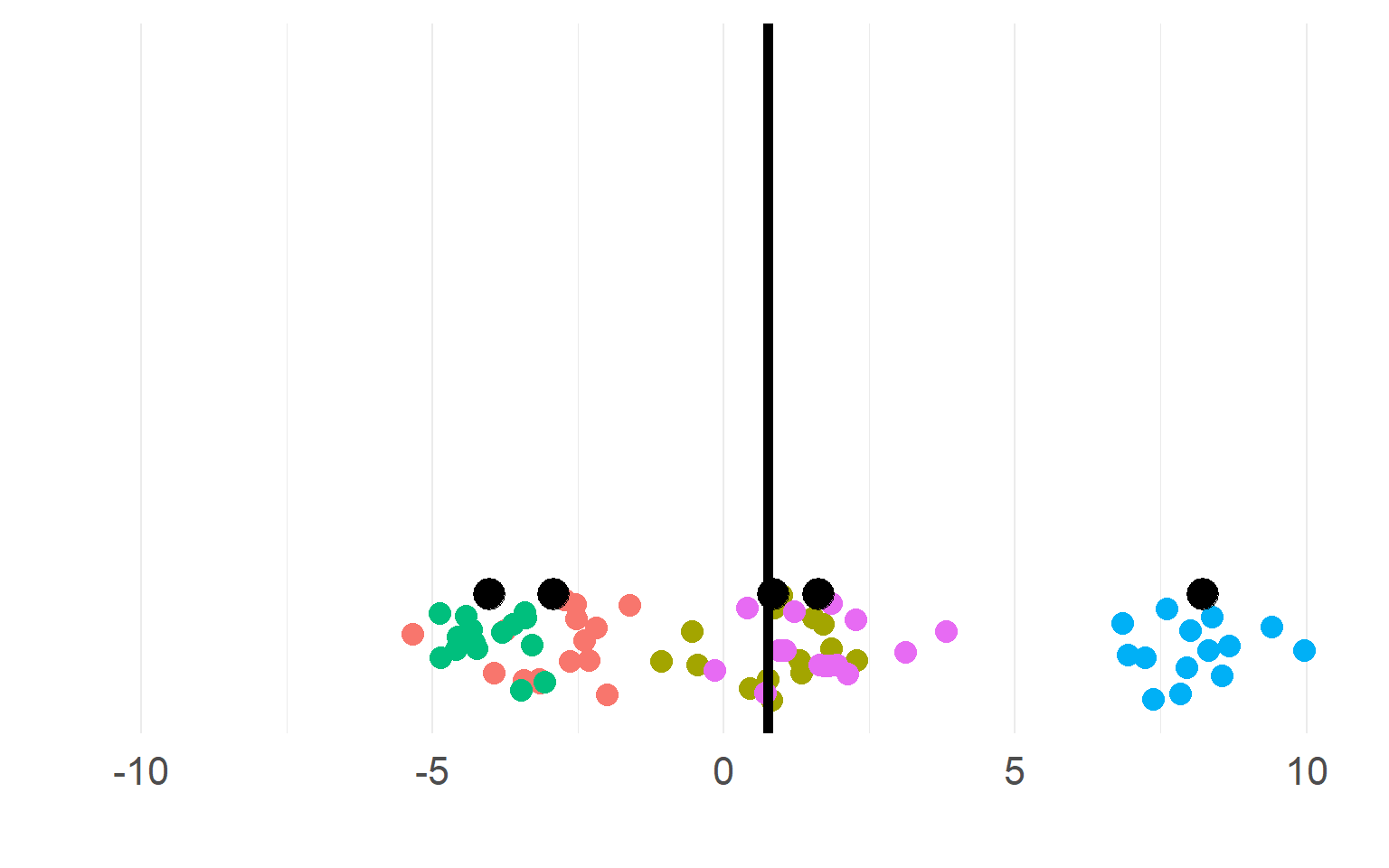with $$n_T =15$$ trials

## Sampling distributions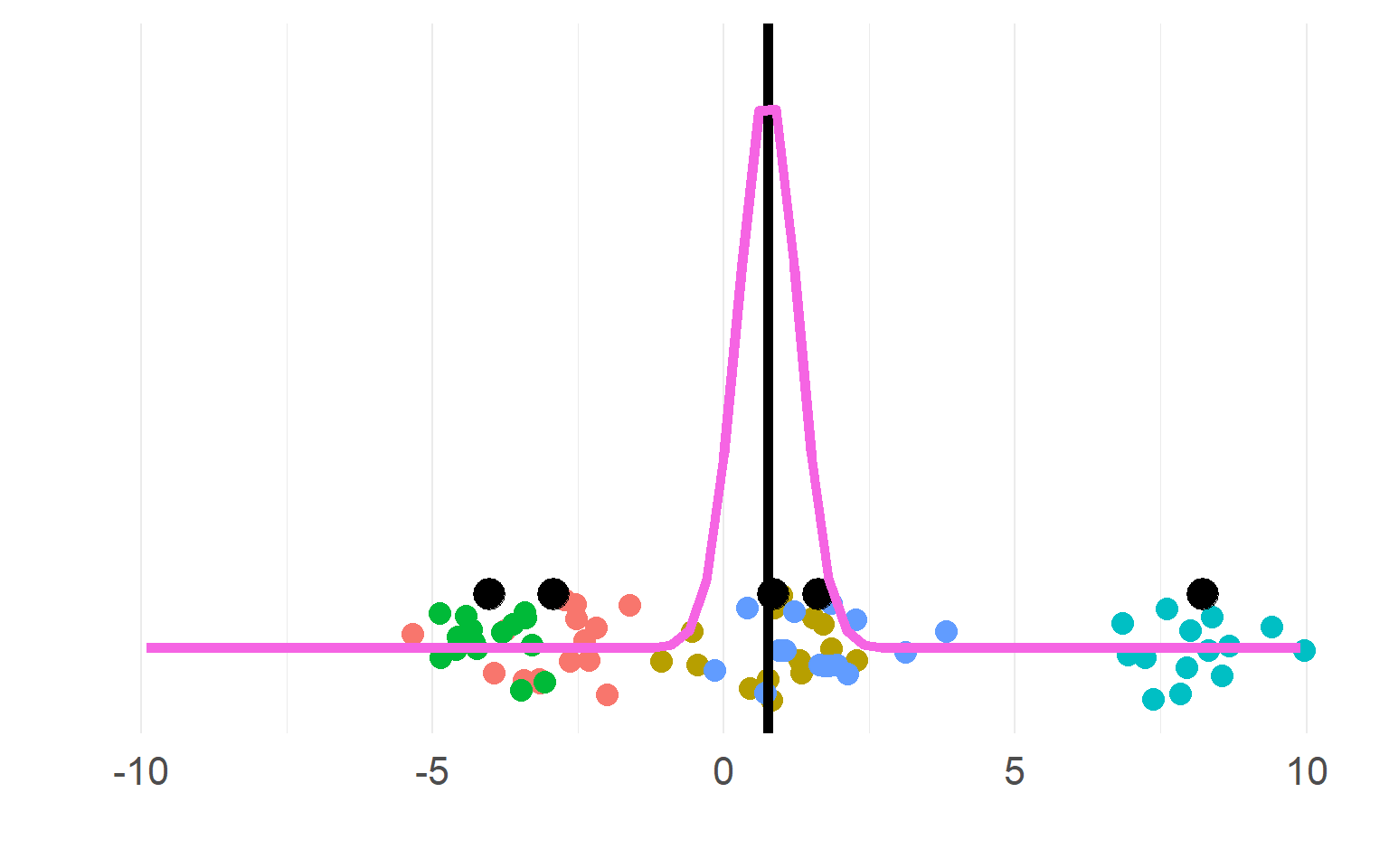This sampling distribution reflects these exact 5 subjects. But does not generalize!

## Sampling distributions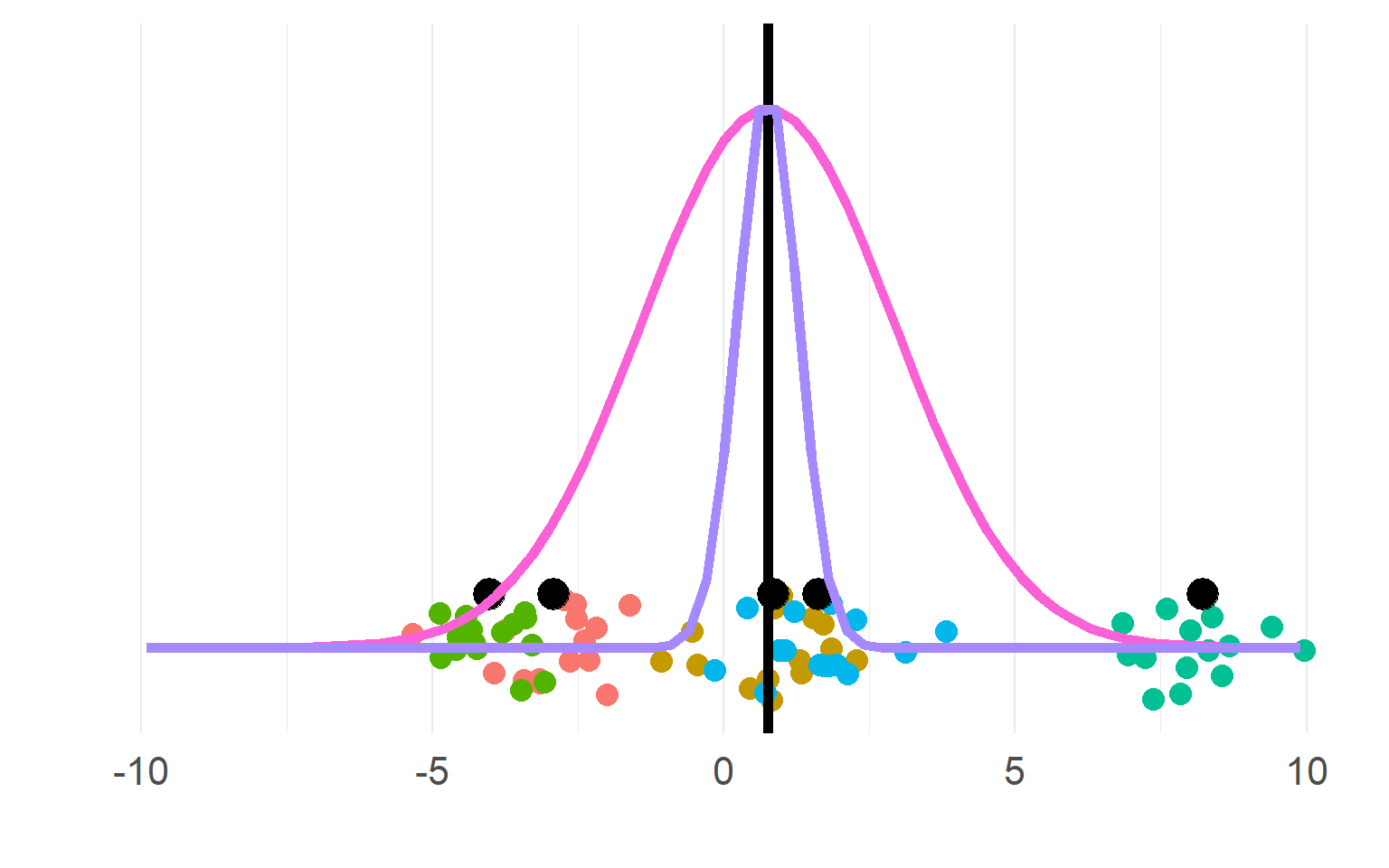(pink for aggregate, purple for disaggregate). There is a strong allure to choose the purple one, easier to get significant results!

$n_{subject}(aggregate) = df+1 = 5$ $n_{"subject"}(pseudoreplication) = df+1= 5*5 = 25$

DONT DO THIS, YOU KNOW (now) BETTER

## Always necessary to aggregate?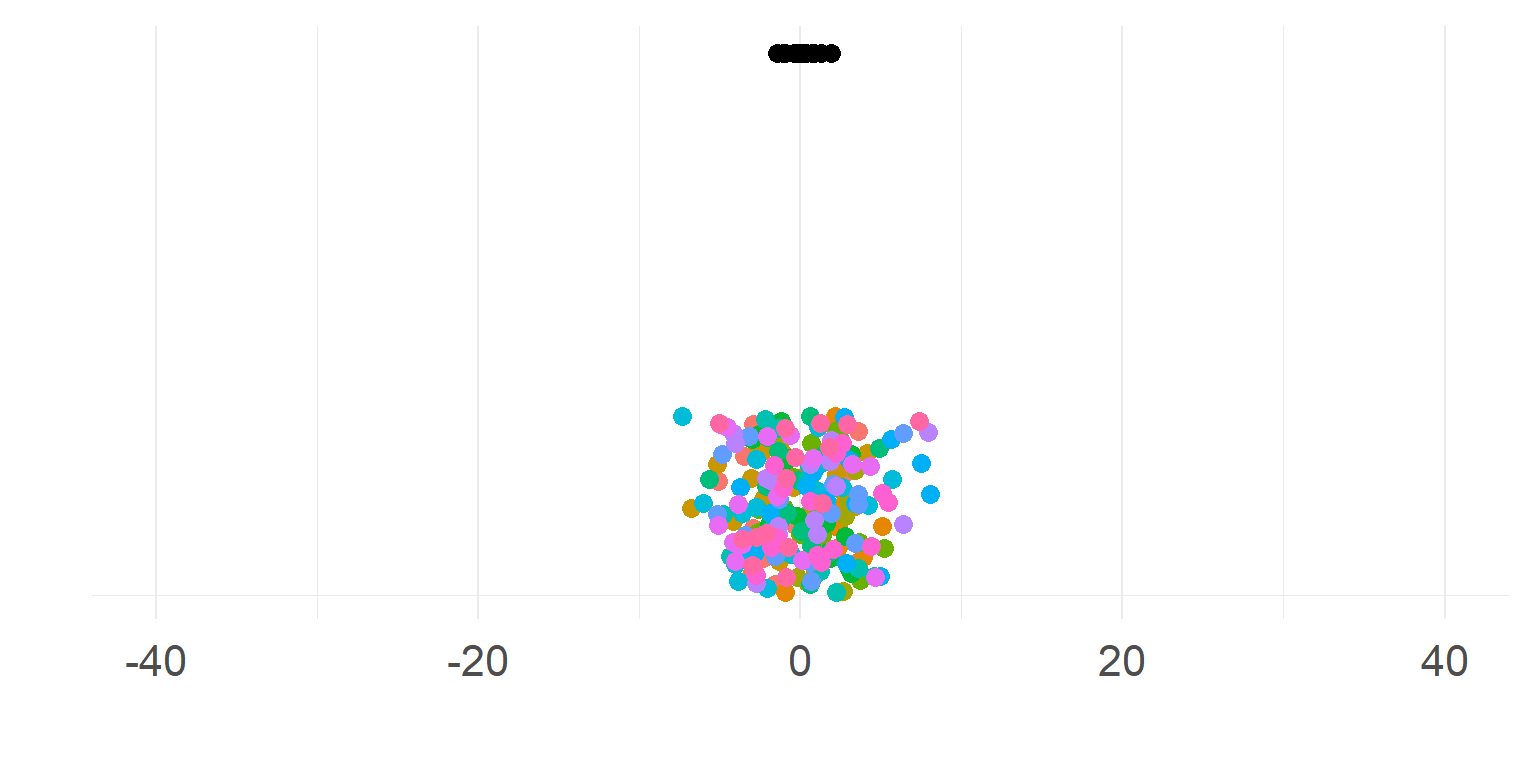$\sigma_{within} >> \sigma_{between}$

Subjects are very similar

Not so necessary to aggregate first

A single parameter summarizes subject-means well

## Should we trust all subjects equally?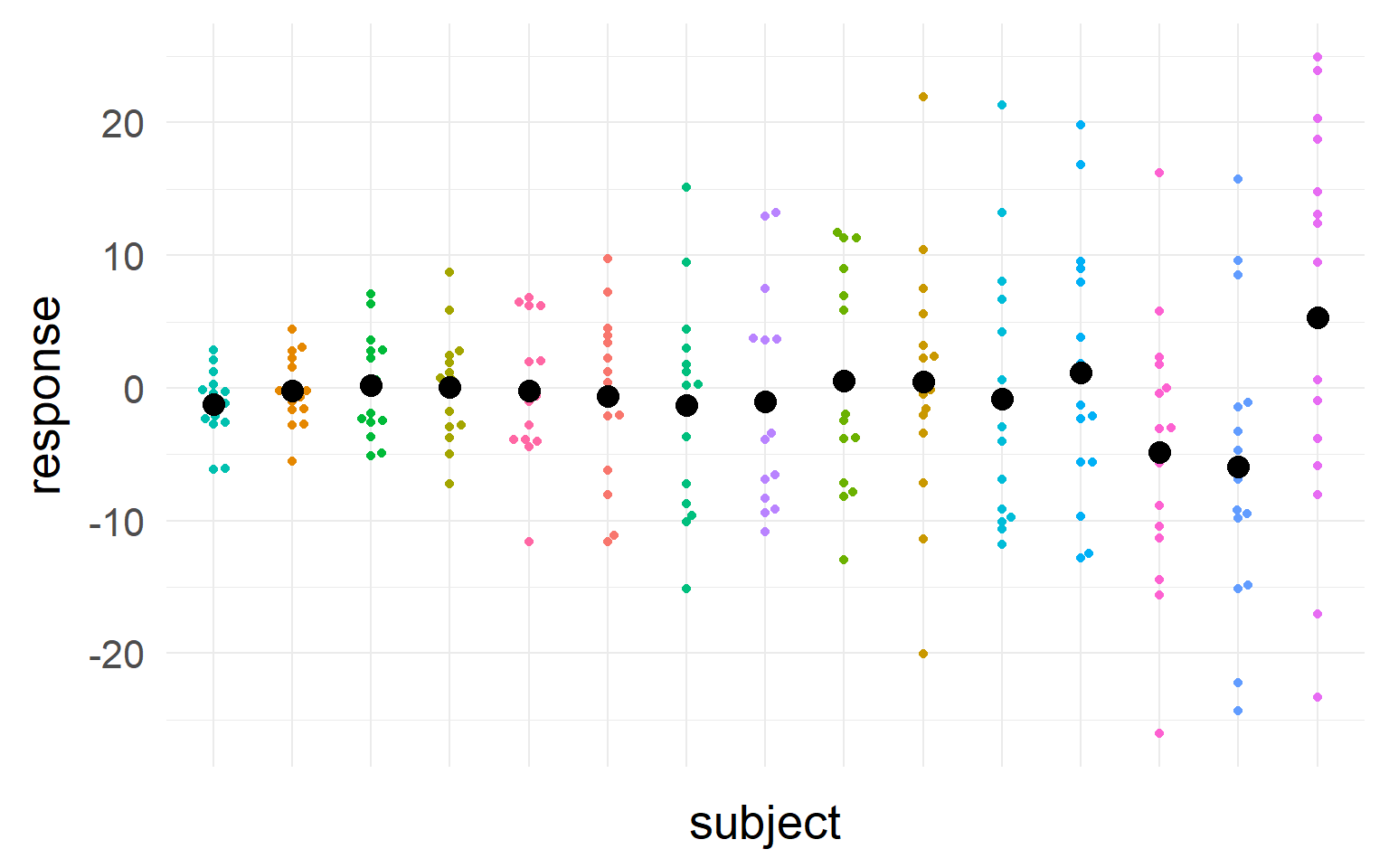Question: Estimate the mean of the population

## Should we trust all subjects equally?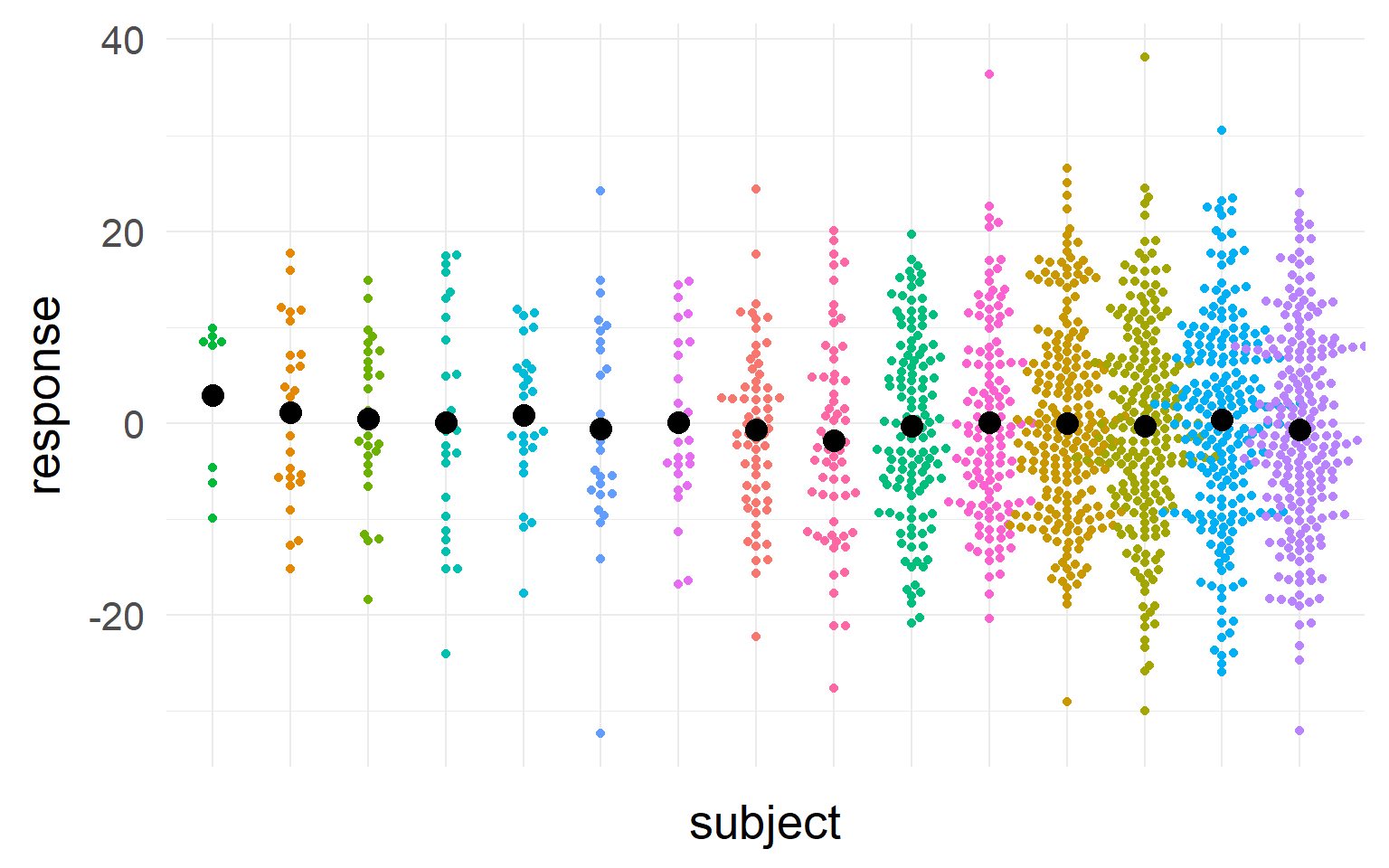Question: Estimate the mean of the population

## Should we trust all subjects equally?We should take into account sample-size and variance into our estimate of the population mean

We should trust those subjects more, where we most sure about their central tendency

## Summary

• Why repeated measures
• More power to find effects that exist!
• Don’t forget about repeated measures!
• Else your type I error will be very high
• Should we trust all subjects equally?
• No, we should punish for small within-subject sample-size and punish high variance

# Overview

• Repeated measures
• An example to guide you through the steps
• The mixed model
• Inference
• Assumption checking
• Shrinkage
• Terminology
• Convergence Problems
• Multiple random variables

## The example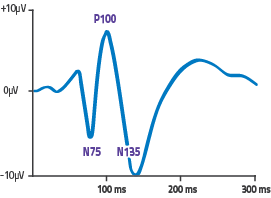EEG-Experiment: Contrast vs. P100 size

=> Very noisy subjects (babys?), between 4 and 29 trials

## The data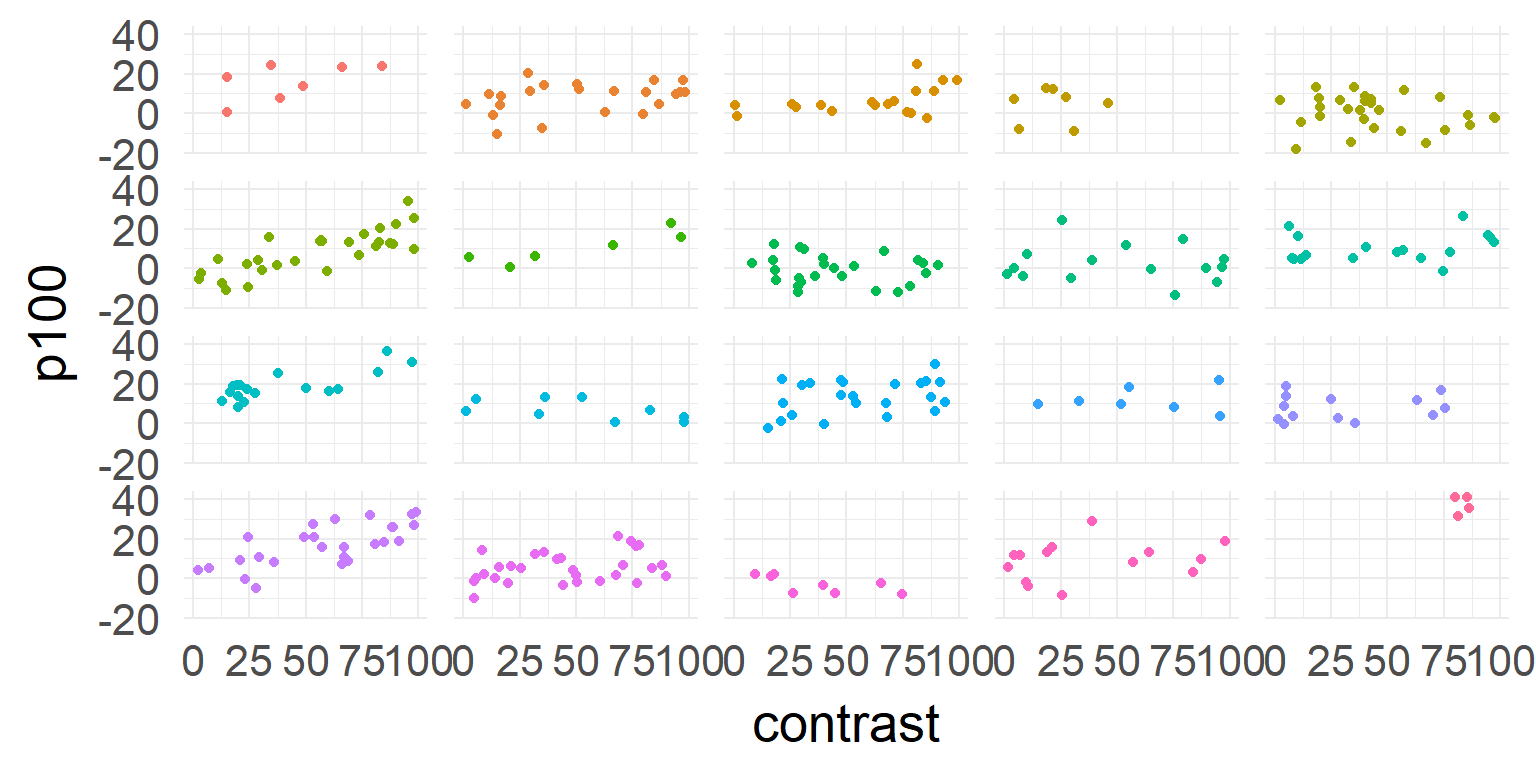## one model for all subjects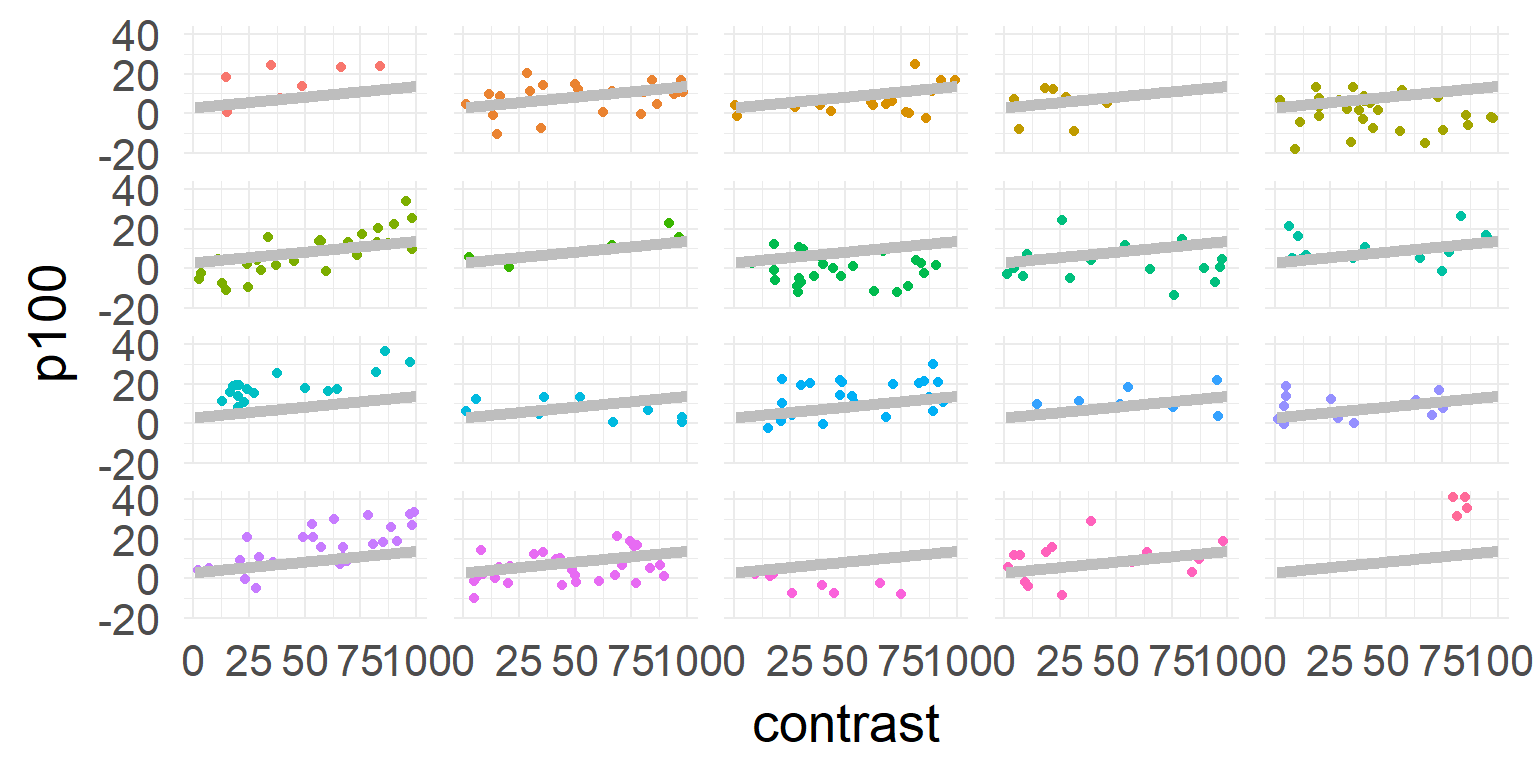$\hat{y} = \beta_0 + \beta_1 * contrast$

## one model for each subject$\hat{y_j} = \beta_{0,j} + \beta_{1,j} * contrast$

## Excourse: two-stage statistics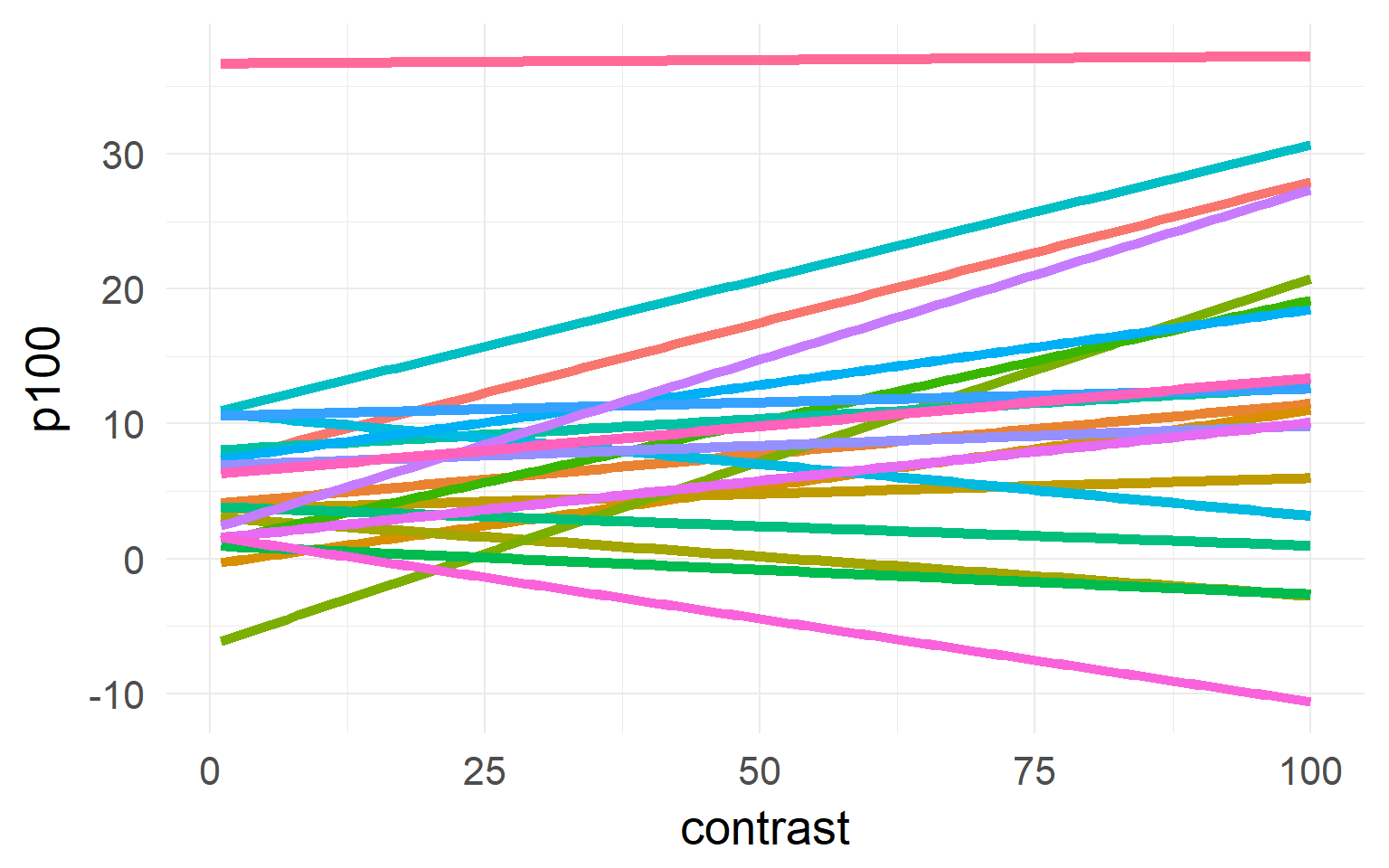## One solutionLinear Mixed Model aka Hierarchical Model aka Multilevel Model

We estimate the population distribution directly. Subject-means with high uncertainty are less influential for the model fit

## A compromiseSmall second level variance (limit $$0$$)

Parameters/DF assumed to be n-trials# Overview

• Repeated measures
• An example to guide you through the steps
• The mixed model
• Inference
• Assumption checking
• Shrinkage
• Terminology
• Convergence Problems
• Multiple random variables

## Formal definition of the linear mixed model## A word of warning

Encouraging psycholinguists to use linear mixed-effects models ‘was like giving shotguns to toddlers’ (Barr)

Because mixed models are more complex and more flexible than the general linear model, the potential for confusion and errors is higher (Hamer)

Many ongoing riddles:

Book:Richly Parameterized Linear Models

• Chp. 9: Puzzles from Analyzing Real Data sets
• Chp.10: A Random Effect Competing with a Fixed Effect
• Chp.12: Competition between Random Effects
• Chp.14: Mysterious, Inconvenient or Wrong Results from Real Datasets

## The mixed model in R

$$\hat{y} = \beta_0 + \beta_1 * contrast + u_{0,j} + u_{1,j} * contrast$$

$$\hat{y}_j = \beta X_j + b_jZ$$

Fitting this in R using the package lme4

response ~ beta + (beta|group)

In our example:

response ~ 1 + contrast + (1|subject) + (0+contrast|subject)

## Mixed models in R

summary(lmer(p100~1 + contrast + (1 + contrast||subject),data=d))
## Linear mixed model fit by REML ['lmerMod']
## Formula: p100 ~ 1 + contrast + ((1 | subject) + (0 + contrast | subject))
##    Data: d
##
## REML criterion at convergence: 2271.4
##
## Scaled residuals:
##      Min       1Q   Median       3Q      Max
## -2.71978 -0.62658 -0.01064  0.65739  2.91110
##
## Random effects:
##  Groups    Name        Variance Std.Dev.
##  subject   (Intercept) 10.16741 3.1886
##  subject.1 contrast     0.01353 0.1163
##  Residual              54.45510 7.3794
## Number of obs: 323, groups:  subject, 20
##
## Fixed effects:
##             Estimate Std. Error t value
## (Intercept)  4.03422    1.10536   3.650
## contrast     0.09536    0.03045   3.131
##
## Correlation of Fixed Effects:
##          (Intr)
## contrast -0.324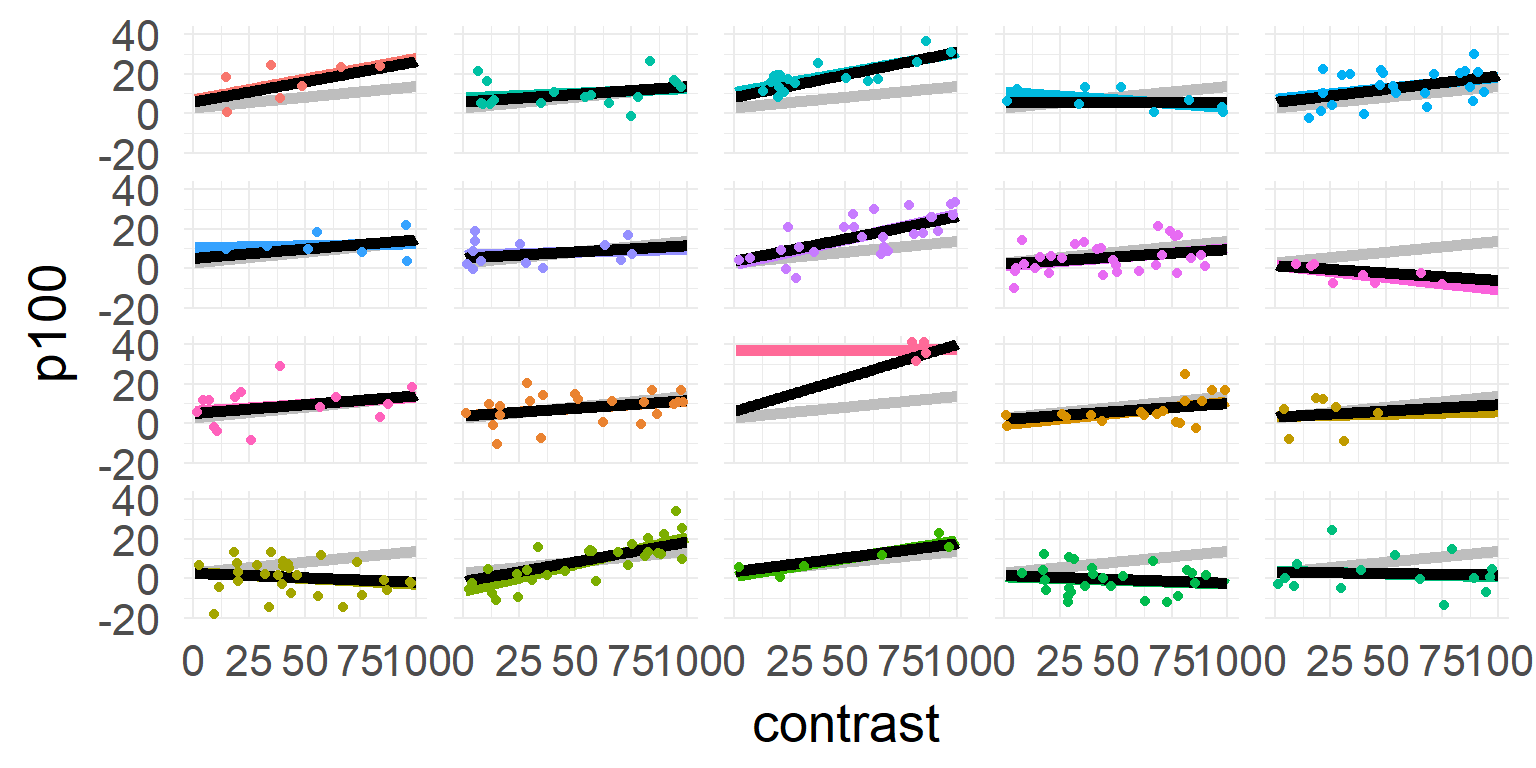gray: one model, color: individual model, black: mixed model

# Overview

• Repeated measures
• An example to guide you through the steps
• The mixed model
• Inference
• REML vs ML
• Assumption checking
• Shrinkage
• Terminology
• Convergence Problems
• Multiple random variables

## Model-Comparisons aka p-values

Unresolved problem!

Read (three links):how to get p values, problems with stepwise regression, different ways to get p-values

One way (good and common, but not the best*): Model comparison for fixed effects:

Be sure to keep random structure equal

anova(mres0,mres1)

“refitting model(s) with ML (instead of REML)”

## Data: d
## Models:
## mres0: p100 ~ 1 + (1 + contrast | subject)
## mres1: p100 ~ 1 + contrast + (1 + contrast | subject)
##       Df    AIC    BIC  logLik deviance  Chisq Chi Df Pr(>Chisq)
## mres0  5 2286.5 2305.3 -1138.2   2276.5
## mres1  6 2280.1 2302.7 -1134.0   2268.1 8.4095      1   0.003733 **
## ---
## Signif. codes:  0 '***' 0.001 '**' 0.01 '*' 0.05 '.' 0.1 ' ' 1

* currently bootstrapping or mcmc recommended

## REML VS ML

### Intuition:

When estimating the variance parameter using maximum likelihood (ML) $$\sigma$$ is biased because $$\mu$$ is not known at the same time

### Solution:

Fit all fixed effects $$\mu$$, then refit the variance parameters on the residing estimates.

This is called restricted maximum likelihood (REML). If $$n$$ is large, no difference between ML and REML exist. If $$n$$ is small REML gives better predictive results.

If you do model comparison and fixed effects (should) differ (usual case) you have to use ML

## Bootstrapping

• resample from the data with replacement (or parametrically from $$H_0$$-Model) 1000 times
• calculate statistic of interest
b1 <- bootMer(mres1, FUN = function(x) as.numeric(logLik(x)), nsim = nboot,seed = 1)
b2 <- bootMer(mres0, FUN = function(x) as.numeric(logLik(x)), nsim = nboot,seed = 1)
bootLL =  2 * b1$t - 2 * b2$t # grab the values & plot
quantile(bootLL, probs = c(0.025, 0.975))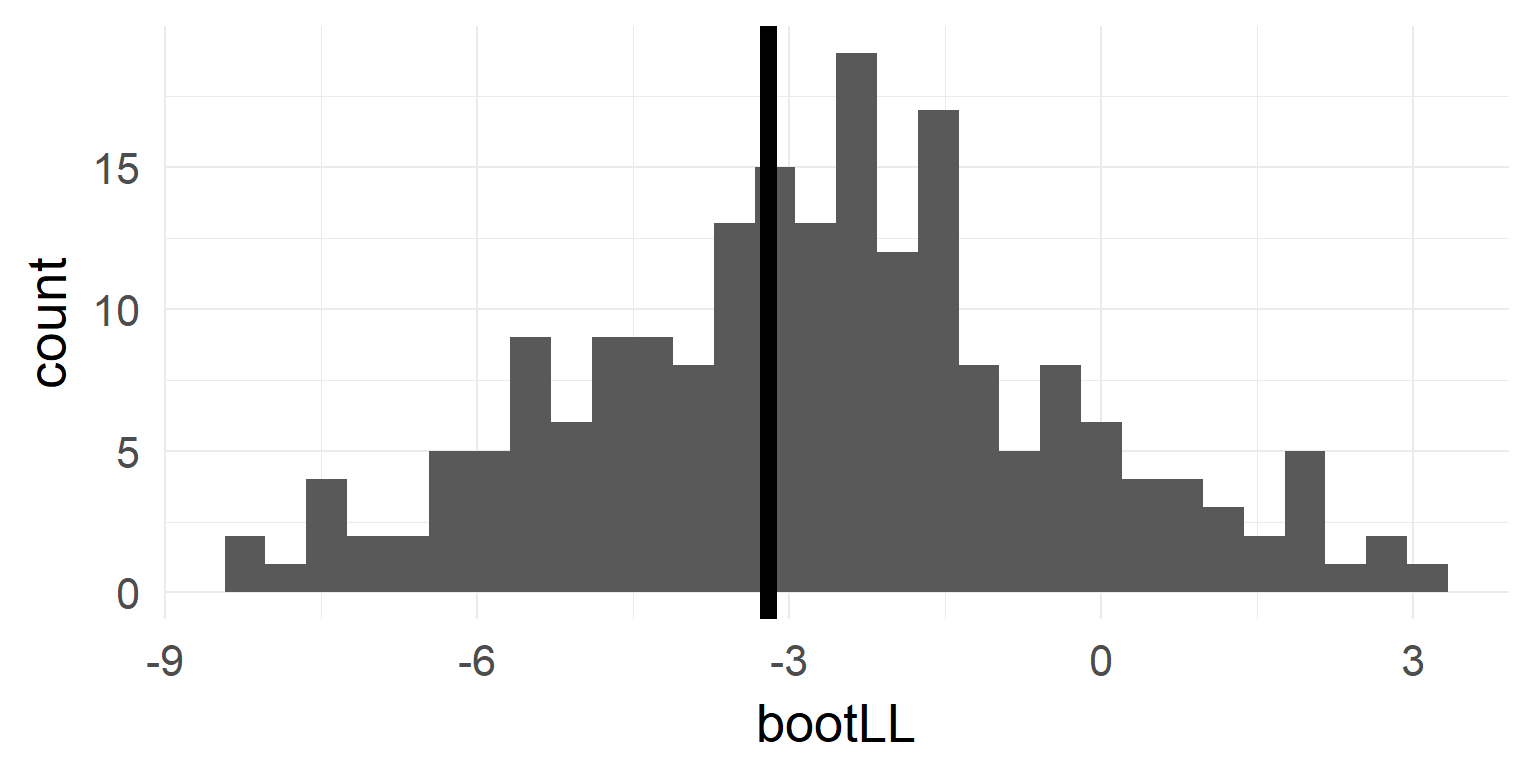## pbkrtest

Better & easier: Likelihood-Ratio using R-Package pbkrtest

summary(pbkrtest::PBmodcomp(mres1,mres0,nsim=nboot))
## Parametric bootstrap test; time: 30.27 sec; samples: 200 extremes: 3;
## large : p100 ~ 1 + contrast + (1 + contrast | subject)
## small : p100 ~ 1 + (1 + contrast | subject)
##            stat     df    ddf  p.value
## PBtest   8.3917               0.019900 *
## Gamma    8.3917               0.011404 *
## Bartlett 7.0365 1.0000        0.007986 **
## F        8.3917 1.0000 12.385 0.013029 *
## LRT      8.3917 1.0000        0.003769 **
## ---
## Signif. codes:  0 '***' 0.001 '**' 0.01 '*' 0.05 '.' 0.1 ' ' 1

The main con against bootstrapping is the high computational time (use at least 1000 not 200 as in this example)

# Overview

• Repeated measures
• An example to guide you through the steps
• The mixed model
• Inference
• Assumption checking
• Level 1
• Level 2
• Shrinkage
• Terminology
• Convergence Problems
• Multiple random variables

## Checking Assumptions Level I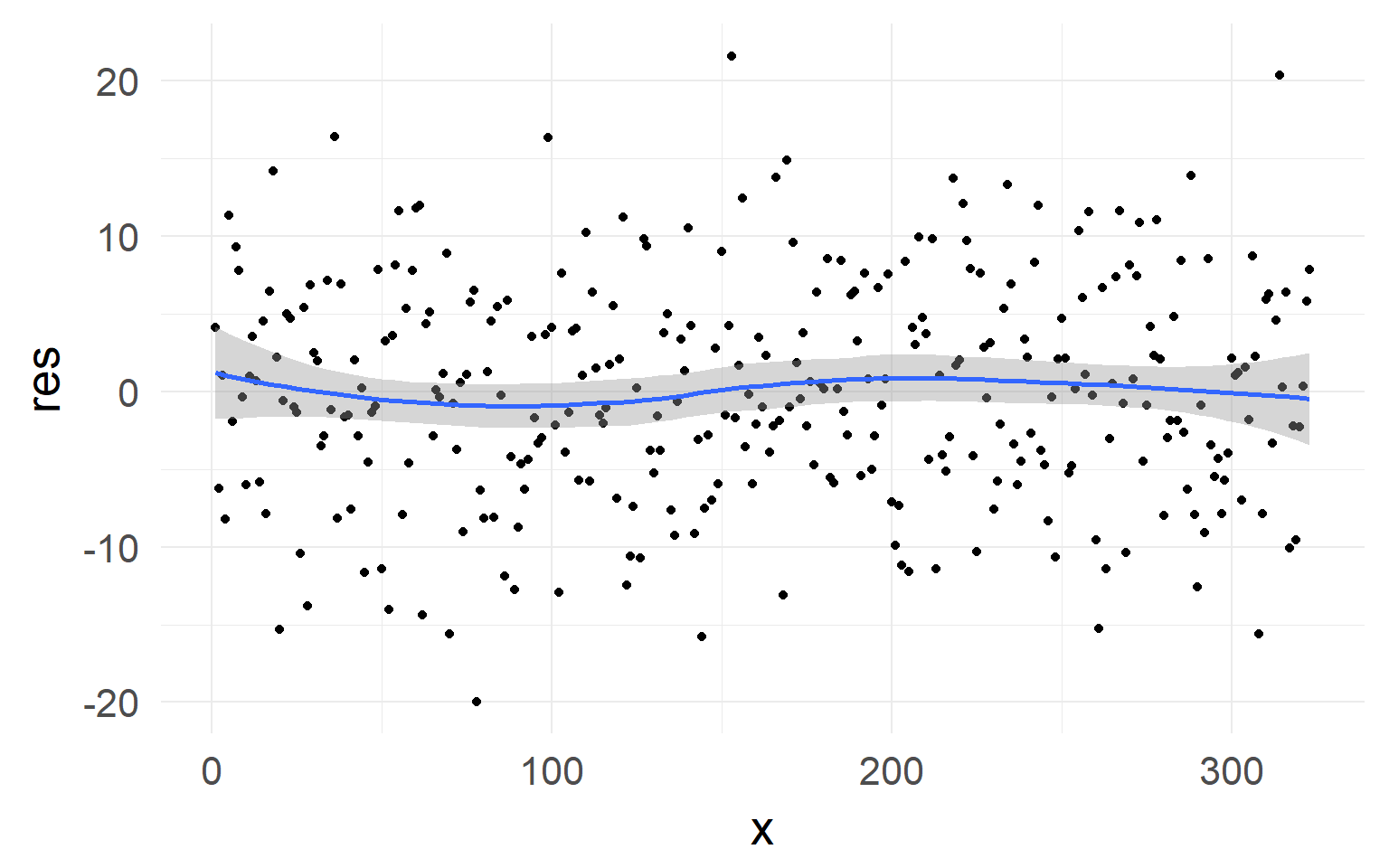No strong non-linearity visible in residuals

## Checking assumptions Level II

In addition: test whether normality for random-variable is true: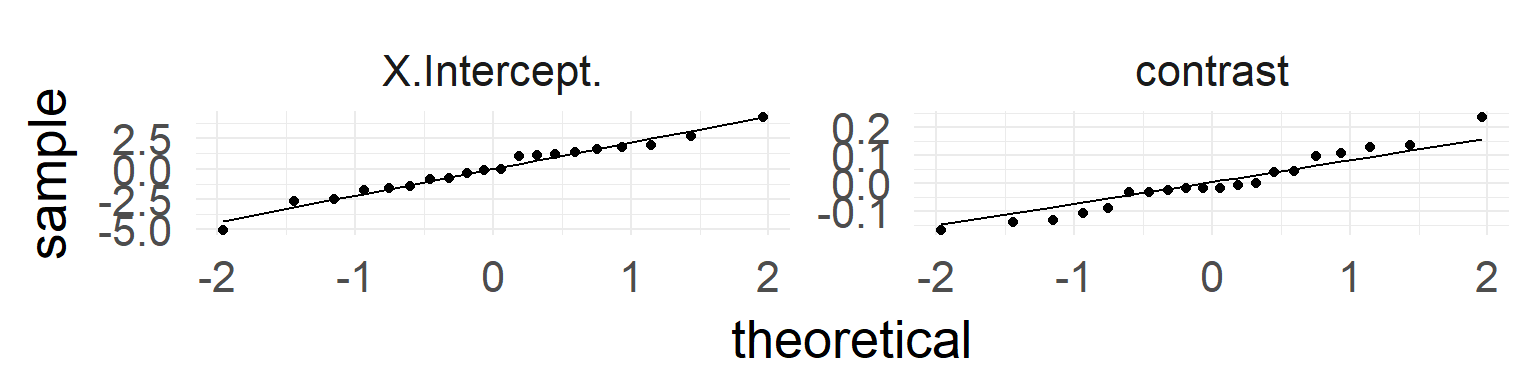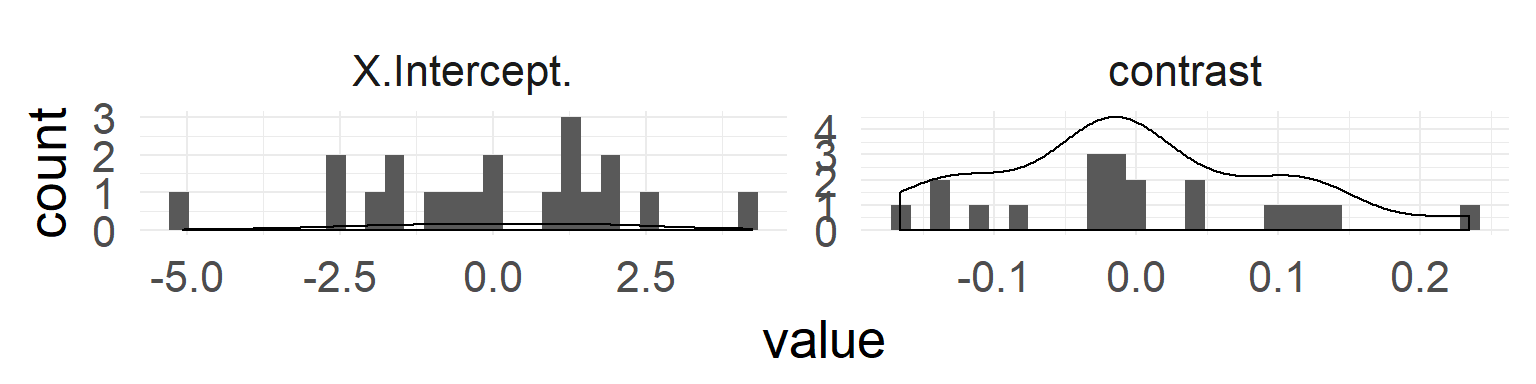QQ-Plots indicate normality of both random effects.

# Overview

• Repeated measures
• The mixed model
• An example to guide you through the steps
• Inference
• Assumption checking
• Shrinkage
• Terminology
• Convergence Problems
• Multiple random variables

## ShrinkageThe individual estimates with high variance / far off the population are shrinked inwards

## Shrinkage-plot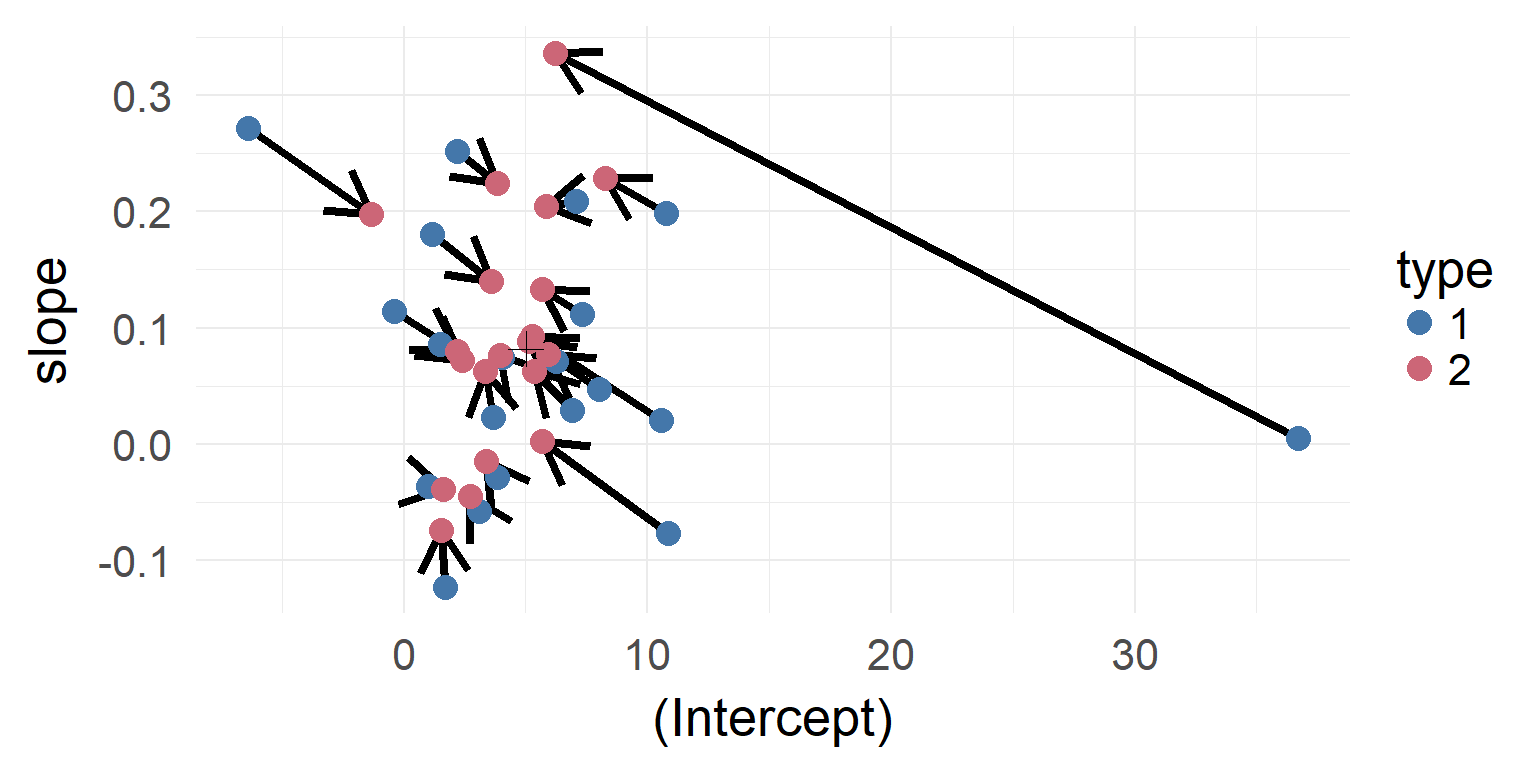gray: one model, color: individual model, black: mixed model (with shrinkage)

## Shrinkage over multiple parameters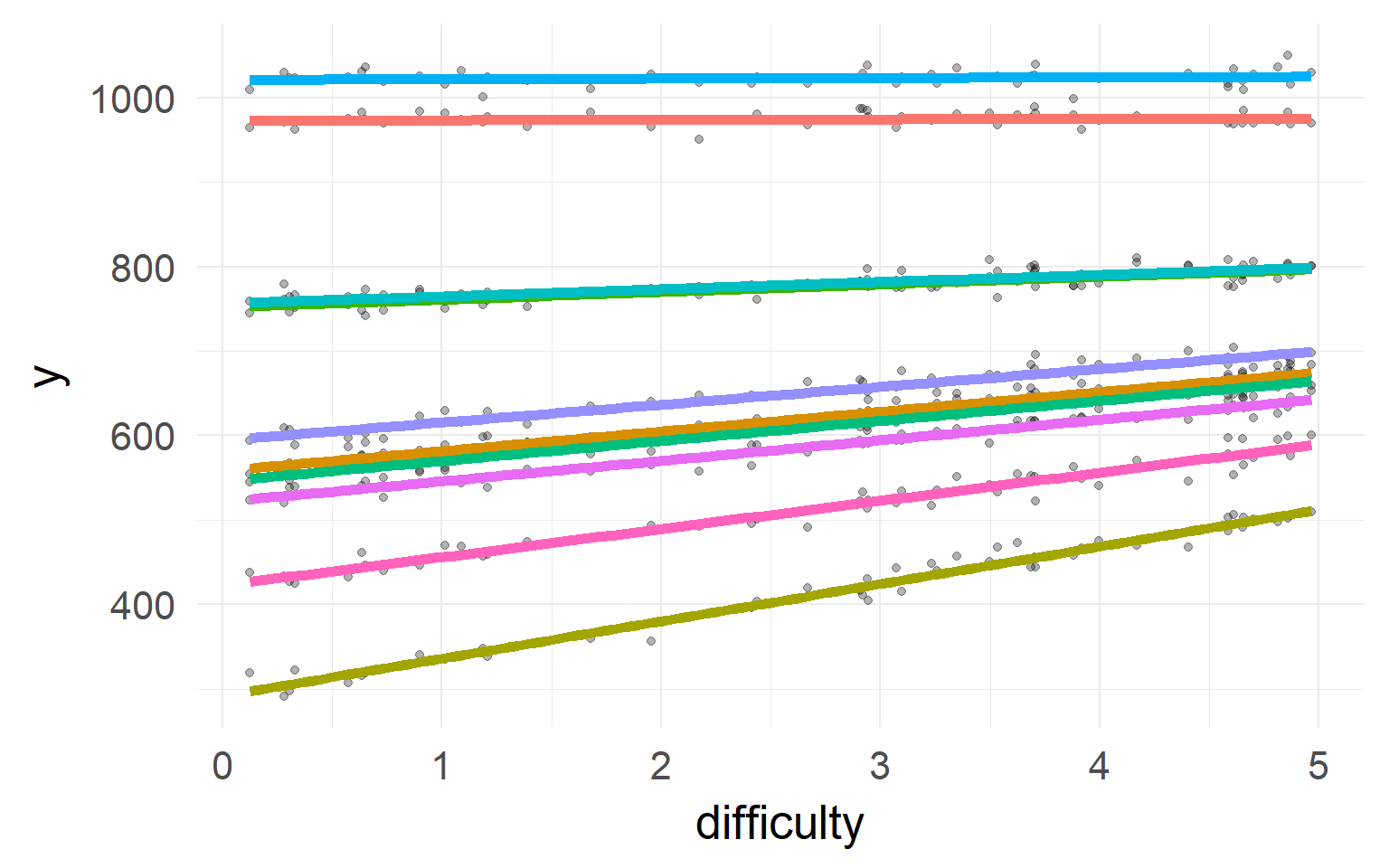Simulated data: Subjects with faster reaction time (intercept) show larger effects for difficulty (slope)

In reverse: If I know somebody is generally slow, he should also have a smaller slope!

## The multivariate normal II$$\rho = 0$$, knowing one dimension does not tell you about the other

$\sum = \left[ {\begin{array}{*{20}{c}} 1&0 \\ 0 &1 \end{array}} \right]$

## Back to mixed models$\sum = \left[ {\begin{array}{*{20}{c}} 1&\rho \\ \rho &1 \end{array}} \right]$

# Overview

• Repeated measures
• The mixed model
• An example to guide you through the steps
• Inference
• Assumption checking
• Shrinkage
• Terminology
• Convergence Problems
• Multiple random variables

## Naming is a bit messy

### Random/Fixed Coefficients

• Random intercept
• (1|subject): Different offset for each subject
• Random slope
• (predictor|subject): Different predictor-slope for each subject
• Random correlation
• (1+predictor|subject) or (X+Y|subject): Knowing about X tells you something about Y
• Fixed coefficient
• exactly the same for all subjects

# Overview

• Repeated measures
• The mixed model
• An example to guide you through the steps
• Inference
• Assumption checking
• Shrinkage
• Terminology
• Convergence Problems
• Multiple random variables

## A more complex model

d$contrastSquare = d$contrast^2
lmer(p100~1+contrast+contrastSquare+(1+contrast+contrastSquare|subject),data = d)


Warning messages:
1: Some predictor variables are on very different scales: consider rescaling
2: In checkConv(attr(opt, "derivs"), opt$par, ctrl = control$checkConv,  :
3: In checkConv(attr(opt, "derivs"), opt$par, ctrl = control$checkConv,  :
Model failed to converge: degenerate  Hessian with 2 negative eigenvalues


## Convergence problems

### Indicator

• Convergence warning (always a problem)
• random effect correlation is either 0 or 1 (very seldom correct)
• model takes very long time to fit (could be correct though)

### Reasons

• misspecified or overspecified models (specified fixed variable as random variable?)
• too many parameters and not enough data,
• data have big outliers/are noisy

• clean data
• center predictors (remove mean) and scale (divide by SD of independent variable)
• use Bayesian fit and put a soft prior on the covariance structure (MCMCglmm,rstanarm,brms)
• simplify the model (parsimoneous models; Bates 2015)

## The ideal case

$\sum = \left[ {\begin{array}{*{20}{c}} 1&{{\rho_{ab}}}&{{\rho_{ac}}}&{{\rho_{ad}}}\\ {{\rho_{ab}}}&1&{{\rho_{bc}}}&{{\rho_{bd}}}\\ {{\rho_{ac}}}&{{\rho_{bc}}}&1&{{\rho_{cd}}}\\ {{\rho_{ad}}}&{{\rho_{bd}}}&{{\rho_{cd}}}&1 \end{array}} \right]$

y ~ 1 + A + B + C + (1 + A + B + C | group)

If you have enough data, try to estimate all correlations.

## The simplest structure

$\Sigma = \left[ {\begin{array}{*{20}{c}} 1&0&0&0\\ 0&1&0&0\\ 0&0&1&0\\ 0&0&0&1 \end{array}} \right]$

y ~ 1 + X1 + X2 + X3 + (1|group) + (0+X1|group) + (0+X2|group) + (0+X3|group)

shorthand:

y ~ 1 + X1 + X2 + X3 + (1+X1+X2+X3||group)

note the || instead if |

## Which one to choose?

• 1: You can estimate the full (enough data or put a lkj-prior if you are Bayes)
• 2: Prior assumption on shape and you don’t have to change it (non-contingent on data)
• 3: Simplify in a data-driven way

Barr promotes full models. Bates promotes a step wise reduction if effects do not significantly explain variance

# Overview

• Repeated measures
• The mixed model
• An example to guide you through the steps
• Inference
• Assumption checking
• Shrinkage
• Terminology
• Convergence Problems
• Multiple random variables

## Multiple random variables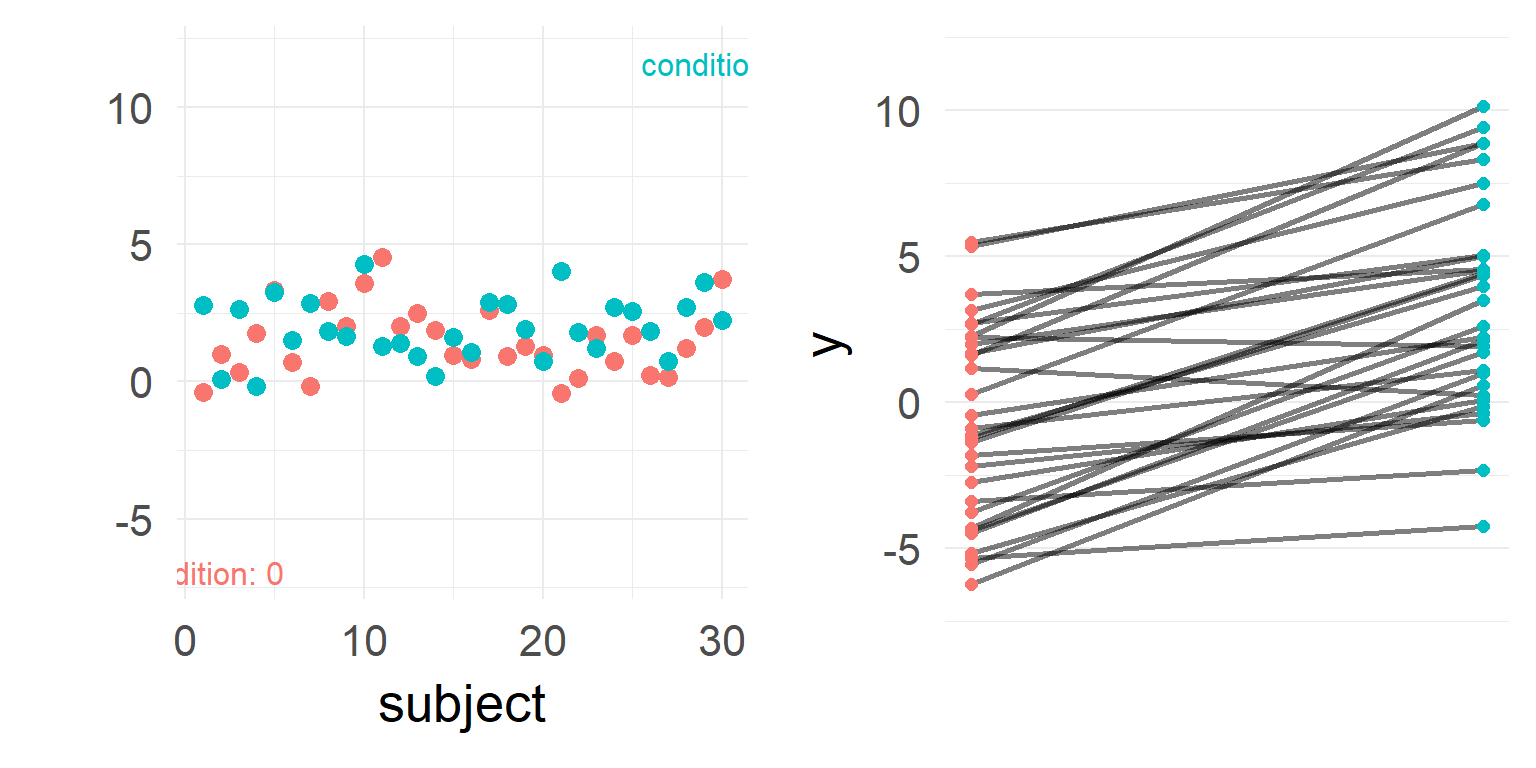What a nice effect! Write paper and end of story?

## But very high stimulus variance!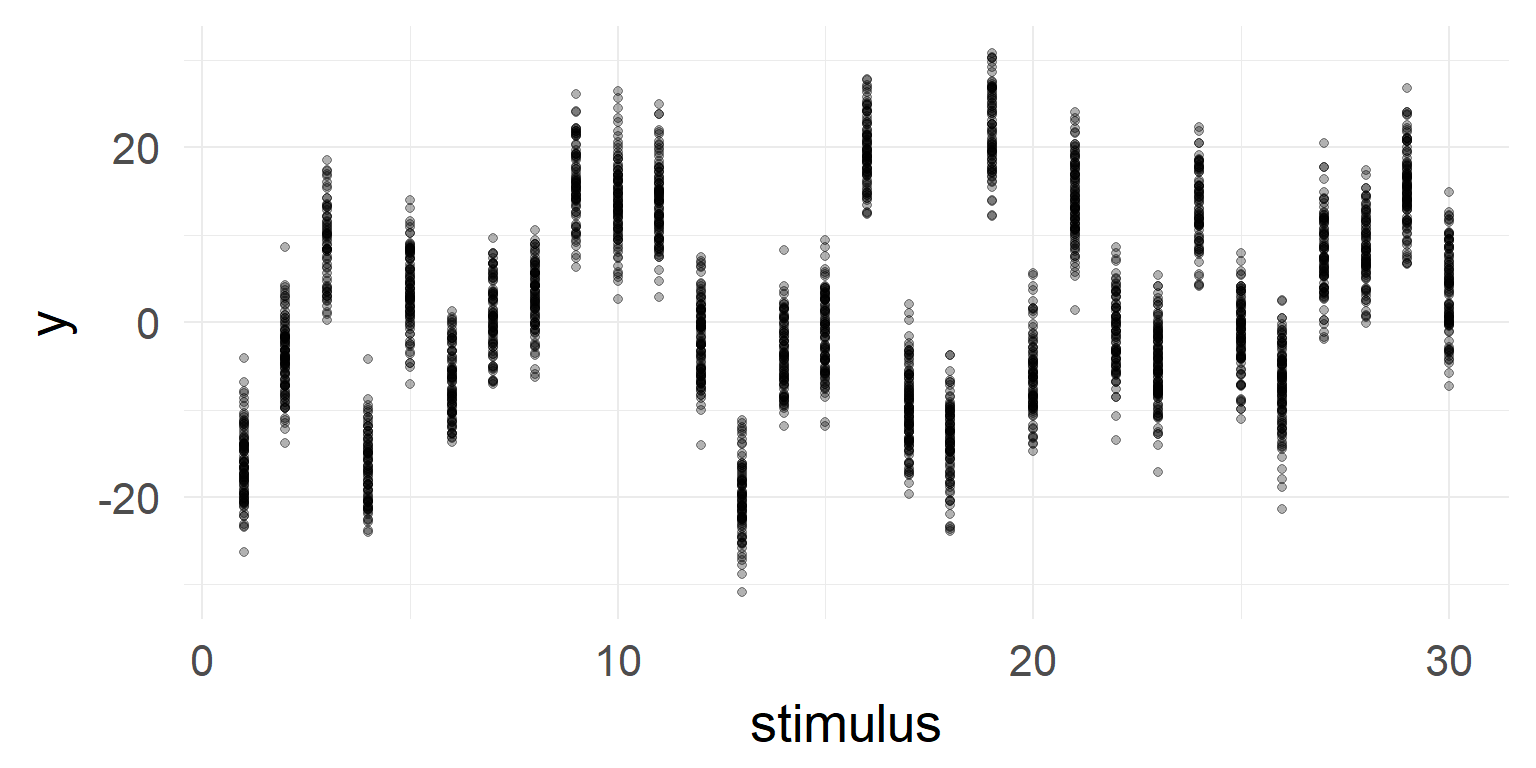## But very high stimulus variance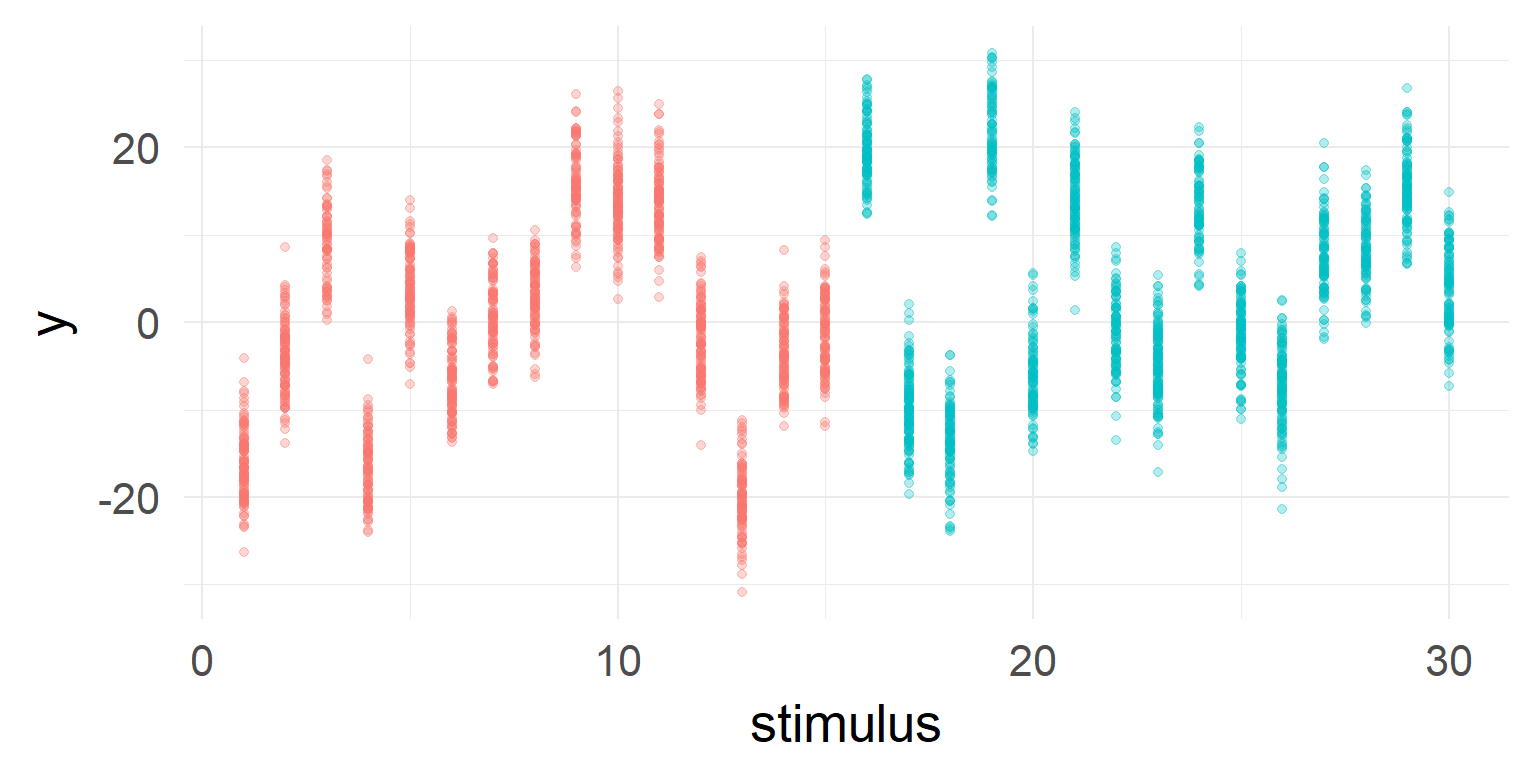## You should include it in your model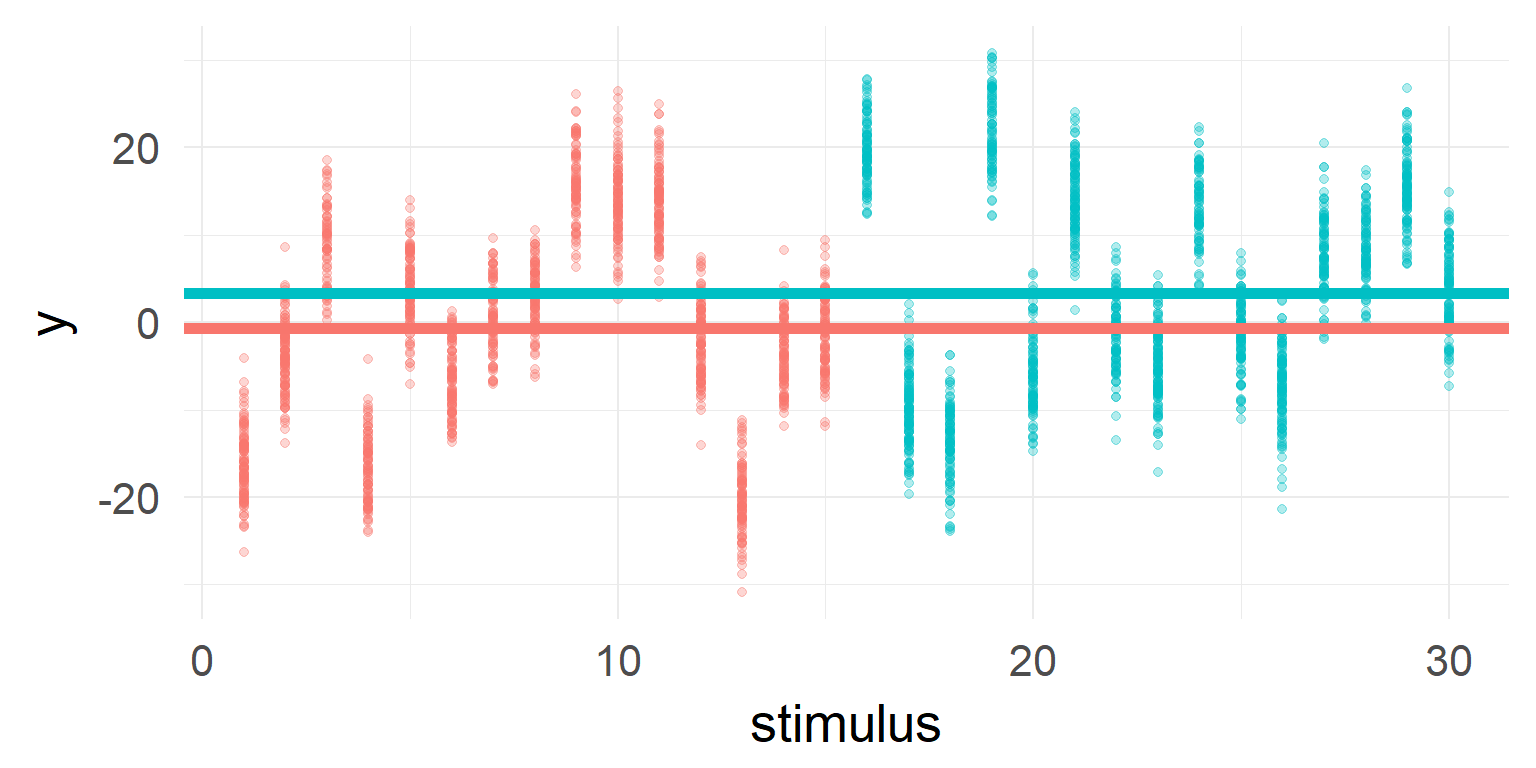Often it is necessary to add multiple random effects! Examples are: subjects, stimuli (images, words, etc)

y ~ 1 + condA (1+condA|subject) + (1+condA|stimulus)

## When to include what

### Some guidance:

• Predictor is a subset of a population?
• => random/grouping variable e.g. subjects, images, words
• Predictor is within subject/grouping?
• => random coefficients
• Predictor is between subject/grouping or aggregated?
• => only fixed coefficient

# Summary

• We learned how to analyze repeated measures data
• We learned how to structure random effects
• We learned how to check assumptions of Mixed Models